# Class 8 RD Sharma Solutions – Chapter 18 Practical Geometry – Exercise 18.4

• Last Updated : 05 May, 2021

### Question 1: Construct a quadrilateral ABCD in which AB = 6 cm, BC = 4 cm, CD = 4 cm, ∠B = 95° and ∠C = 90°?

Solution:

We have, AB = 6 cm, BC = 4 cm, CD = 4 cm, ∠B = 95° and ∠C = 90°.

Hey! Looking for some great resources suitable for young ones? You've come to the right place. Check out our self-paced courses designed for students of grades I-XII

Start with topics like Python, HTML, ML, and learn to make some games and apps all with the help of our expertly designed content! So students worry no more, because GeeksforGeeks School is now here!

Step 1: Draw a line segment BC = 4cm

Step 2: Construct an angle of 95° at B.

Step 3: Taking B as the centre, draw an arc radius of 6 cm. Mark this point as A.

Step 4: Construct an angle of 90° at C.

Step 5: Taking C as the centre, draw an arc radius of 4 cm. Mark this point as D.

Step 6: Now join the line segments BA, CD, and AD to form a quadrilateral.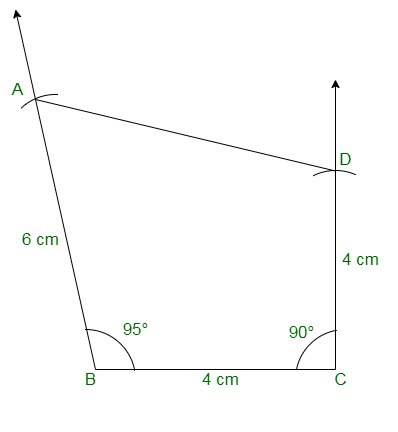### Question 2: Construct a quadrilateral ABCD where AB = 4.2cm, BC = 3.6 cm, CD = 4.8 cm, ∠B = 30° and ∠C = 150°?

Solution:

The given details are AB = 4.2cm, BC = 3.6 cm, CD = 4.8 cm, ∠B = 30° and ∠C = 150°.

Step 1: Draw a line segment BC = 3.6cm

Step 2: Construct an angle of 30° at B.

Step 3: Taking B as the centre, draw an arc radius of 4.2 cm. Mark this point as A.

Step 4: Construct an angle of 150° at C.

Step 5: Taking C as the centre, draw an arc radius of 4.8 cm. Mark this point as D.

Step 6: Now join BA, CD, and AD to form a quadrilateral.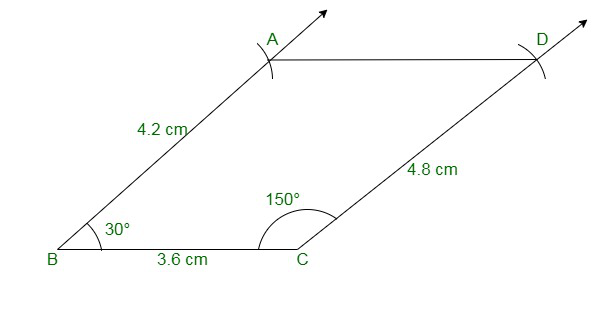### Question 3: Construct a quadrilateral PQRS in which PQ = 3.5 cm, QR = 2.5 cm, RS = 4.1 cm, ∠Q = 75° and ∠R = 120°?

Solution:

The given details are PQ = 3.5 cm, QR = 2.5 cm, RS = 4.1 cm, ∠Q = 75° and ∠R = 120°.

Step 1: Draw a line segment QR = 2.5cm

Step 2: Construct an angle of 75° at Q.

Step 3: Taking Q as the centre, draw an arc radius of 3.5 cm. Mark this point as P.

Step 4: Construct an angle of 120° at R.

Step 5: Taking R as the centre, draw an arc radius of 4.1 cm. Mark this point as S.

Step 6: Now join QP, RS, and PS to form a quadrilateral.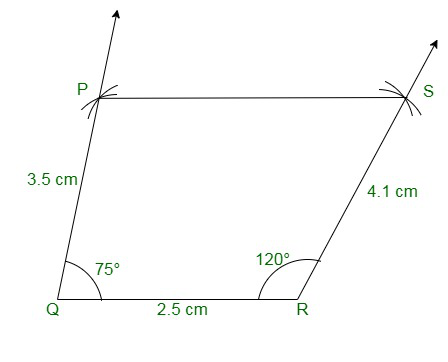### Question 4: Construct a quadrilateral ABCD given BC = 6.6 cm, CD = 4.4 cm, AD = 5.6 cm ∠D = 100° and ∠C = 95?

Solution:

We have, BC = 6.6 cm, CD = 4.4 cm, AD = 5.6 cm ∠D = 100° and ∠C = 95

Step 1: Draw a line segment DC = 4.4cm

Step 2: Construct an angle of 100° at D.

Step 3: Taking D as the centre, draw an arc radius of 5.6 cm. Mark this point as A.

Step 4: Construct an angle of 95° at C.

Step 5: Taking C as the centre, draw an arc radius of 6.6 cm. Mark this point as B.

Step 6: Now join DA, CB, and AB to form a quadrilateral.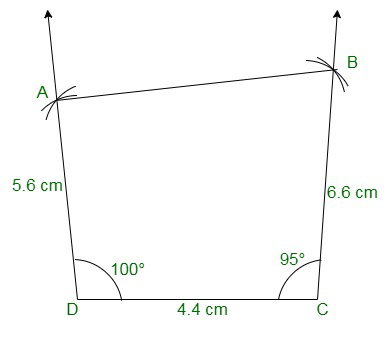### Question 5: Construct a quadrilateral ABCD in which AD = 3.5 cm, AB = 4.4 cm, BC = 4.7 cm, ∠A = 125° and ∠B = 120°?

Solution:

The given details are AD = 3.5 cm, AB = 4.4 cm, BC = 4.7 cm, ∠A = 125° and ∠B = 120°.

Step 1: Draw a line AB = 4.4cm

Step 2: Construct an angle of 125° at A.

Step 3: Taking A as the centre, draw an arc radius of 3.5 cm. Mark this point as D.

Step 4: Construct an angle of 120° at B.

Step 5: Taking B as the centre, draw an arc radius of 4.7 cm. Mark this point as C.

Step 6: Now join AD, BC, and CD to form a quadrilateral.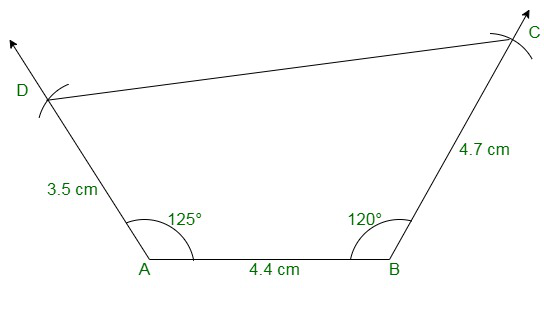### Question 6: Construct a quadrilateral PQRS in which ∠Q = 45° and ∠R = 90°, QR = 5 cm, PQ = 9 cm and RS = 7 cm?

Solution:

We have, ∠Q = 45° and ∠R = 90°, QR = 5 cm, PQ = 9 cm and RS = 7 cm.

Step 1: Draw a line segment QR = 5cm

Step 2: Construct an angle of 45° at Q.

Step 3: Taking Q as the centre, draw an arc radius of 9 cm. Mark this point as P.

Step 4: Construct an angle of 90° at R.

Step 5: Taking R as the centre, draw an arc radius of 7 cm. Mark this point as S.

Step 6: Now join the line segments QP, RS.

Since the line segment, QP and RS are not intersecting at each other, a quadrilateral cannot be formed.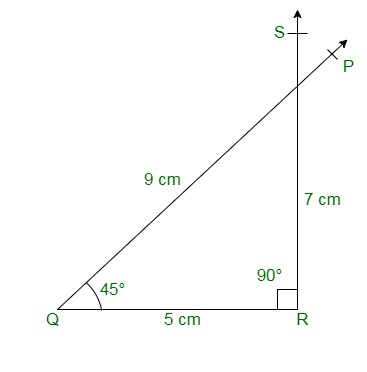### Question 7: Construct a quadrilateral ABCD in which AB = BC = 3 cm, AD = 5 cm, ∠A = 90° and ∠B = 105°?

Solution:

We have, AB = BC = 3 cm, AD = 5 cm, ∠A = 90° and ∠B = 105°.

Step 1: Draw a line AB = 3cm

Step 2: Construct an angle of 90° at A.

Step 3: Cut an arc of radius 5cm with A as the centre to mark that point as D.

Step 4: Construct an angle of 105° at B.

Step 5: Cut an arc of radius 3cm with B as the centre to mark that point as C.

Step 6: Now join AD, BC, and CD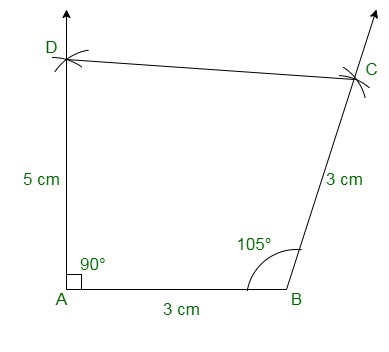### Question 8: Construct a quadrilateral BDEF, where DE = 4.5 cm, EF = 3.5 cm, FB = 6.5 cm, ∠F = 50° and ∠E = 100°?

Solution:

We have, DE = 4.5 cm, EF = 3.5 cm, FB = 6.5 cm, ∠F = 50° and ∠E = 100°.

Step 1: Draw a line EF = 3.5cm

Step 2: Construct an angle of 100° at E.

Step 3: Cut an arc of radius 4.5cm with E as the centre to mark that point as D.

Step 4: Construct an angle of 50° at F.

Step 5: Cut an arc of radius 6.5cm with F as the centre to mark that point as B.

Step 6: Now join DE, FB, and DB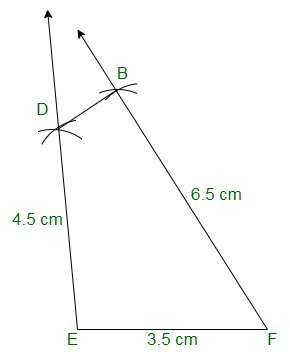My Personal Notes arrow_drop_up